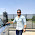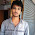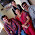As we know, questions related to number series are very important in Quantitative aptitude section, So, today I'm going to discuss some problems of number series. These are just for your practice. I have already discussed this chapter in previous session i.e. Sequence and Series. Read this article first, then go through these examples.

## Examples with Solutions

Example1: In the following given series, find out the wrong number.

1, 3, 10, 21, 64, 129, 356

Solution: As i have discussed in previous session, operations applied on series can be: Addition or subtraction, multiplication or division, squaring or cubing and combination of any.

Series                 1        3       10       21      64       129          356
Difference               2        7       11       43       65         227

By studying the above series, you'll come to know that, this is a combination of operations. Operation used is discussed as follows:

Alternately using: (times 2 + 1) and (times 3 + 1)

1 times 2 + 1 = 3
3 times 3 + 1 = 10
10 times 2 + 1 = 21
21 times 3 + 1 = 64
64 times 2 + 1 = 129
129 times 3 + 1 = 388

Therefore, last number of series is wrong i.e.356

Example2: Find the next number of the following series:

672, 560, 448, 336, 224,?

Solution: Check out the difference of each number first:

Series:           672      560     448     336     224
Difference:         112      112      112    112

Difference between all the terms is same i.e. 112

Therefore, next term will be = 224 - 112 = 112 Ans

Example3: Find the next term of following series:
82, 67, 54, 43, 34

Solution: The operation used in series is discussed as follow:
9^2 + 1 = 82
8^2 + 3 = 67
7^2 + 5 = 54
6^2 + 7 = 43
5^2 + 9 = 34

Continuing like this;

4^2 + 11 = 27

Therefore, 27 is the next term.

Example4: What should come in place of question mark?

1721, 2190, 2737, 3368,?

Solution: As we can see, first term is approximately equal to the cube of 12, so firstly we will try to solve it with the cubes.

(12)^3 - 7 = 1721
(13)^3 - 7 = 2190
(14)^3 - 7 = 2737
(15)^3 - 7 = 3368

Now you can find, that there is some pattern. So, continuing like this, we get
(16)^3 - 7 = 4089 Ans

Example5: Find the next term of following series:

8, 24, 12, 36, 18, ?

Solution: Relation between the terms is as follows:

8 times 3= 24

(24/2) = 12

12 times 3=36

(36)/2=18

Continuing like this:
18 times 3 = 54

Therefore, next term is 54

Example 6: Find the next term of the following series:

47, 33, 21, 11, ?

Solution: The pattern used in the series is as follows:

7^2 - 2 = 47
6^2 - 3 = 33
5^2 - 4 = 21
4^2 - 5 = 11
By continuing, we get
3^2 - 6 = 3
Therefore, 3 is the next term.

I will soon update the questions for your practice.
Solved examples of number series in Quantitative aptitudeReviewed by Jasleen Behl on Monday, January 20, 2014 Rating: 5

1.Nice 1... Really Healpful

2.thanx

3.superb

4.SUMANTH KUMAR REDDYJanuary 11, 2015 at 8:45 PM

nice sir

5.what is next number in this series 11 19 13 21 17 22 25

•23

•23

•i got 33

•xx , 19 , xx , 19+2=21 , xx , 21+1=22 , xx , [22+0=22]

6.abhishek bhardwajOctober 3, 2015 at 9:23 PM

1,7,12,?,45,147 with solution

7.22.5,41

8.Thanks....

9.Thank you....

I will try to respond asap